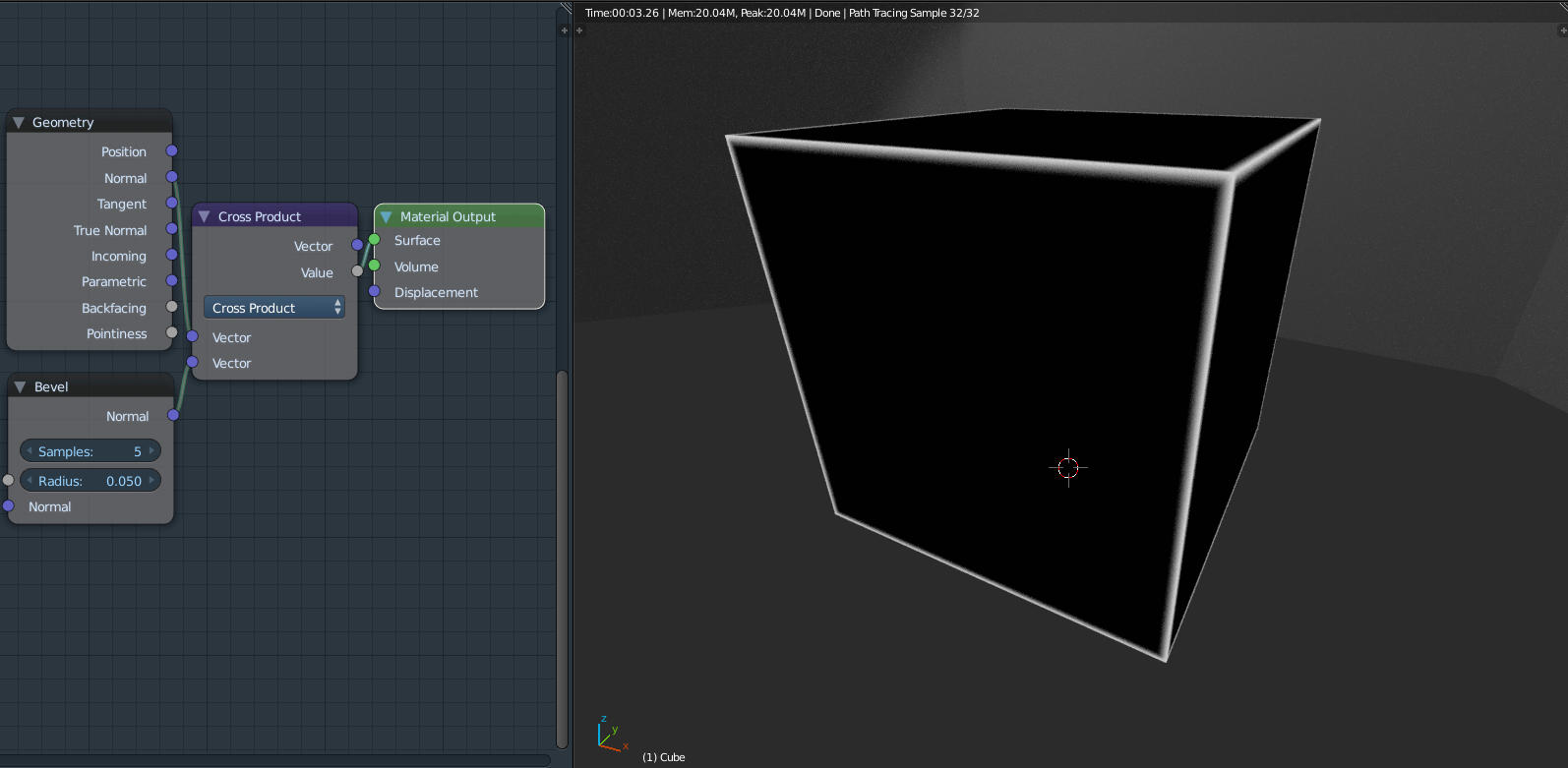# Shading sharp edges in Cycles with the Bevel node

The Bevel shader node for Cycles appears to be a Blender 2.8 feature that is present in Blender 2.79 development builds.

This node is handy for shading sharp edges differently from smooth parts of a model.

Its output is the same as that of the Normal output of the Geometry node, except at edges, where the normals are modified to give the effect of a slight bevel toward the edge for shading purposes, without having to model this.

Geometry node normals:Bevel node normals:Sharp edges can be isolated by using a vector operation that is only non-zero when the “bevelled” normals differ from the original ones. Two operations that fulfil that criterion are Vector Math -> Cross Product and Vector Math -> Subtract.

Here is the result of taking the Cross Product and using its Value, which is zero when the two normals are aligned, as a surface shader:To make the edge effect adjustable in intensity, I added a Multiply Math node after the Cross Product, and then I made a node group out of it to tidy up the workspace.Now the node group (which I called GetEdges) can be used as the mix factor to combine two shaders, using the Mix Shader – one for the flat surfaces and one for the edges.

Here’s an example for a transparent object with glowing edges:Interesting effects can be made with more complex and smoother models as well, but they will look less like wireframes. Here are two monkeys, one shaded smooth and one shaded flat, using the same variation of the above edge-detecting emission shader, as an illustration.